Courses

# Test: Measurement Length, Weight And Capacity

## 20 Questions MCQ Test Mathematics for Class 5 (V) - CBSE and NCERT Curriculum | Test: Measurement Length, Weight And Capacity

Description
This mock test of Test: Measurement Length, Weight And Capacity for Class 5 helps you for every Class 5 entrance exam. This contains 20 Multiple Choice Questions for Class 5 Test: Measurement Length, Weight And Capacity (mcq) to study with solutions a complete question bank. The solved questions answers in this Test: Measurement Length, Weight And Capacity quiz give you a good mix of easy questions and tough questions. Class 5 students definitely take this Test: Measurement Length, Weight And Capacity exercise for a better result in the exam. You can find other Test: Measurement Length, Weight And Capacity extra questions, long questions & short questions for Class 5 on EduRev as well by searching above.
QUESTION: 1

Solution:
QUESTION: 2

Solution:
QUESTION: 3

### Soni can cycle from point A to point B and return to point A in 10 minutes. She can cycle and walk back in 18 minutes. It will take ___ mins for her to walk there and walk back.

Solution:

Let time for cycling =x and time for walking = y
We havex+x = 10,x+y = 182x = 10 x = 5
∴ y = 13 Hence time in walking from both sides = 26 minutes.

QUESTION: 4

Rajesh bought 13 kg of peanuts. He saved? 195 by using a store coupon. How much did he save per kg of ground nuts?

Solution: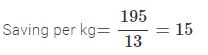QUESTION: 5

Julie has Rs. 61.50 to buy lunch. If she buys a burger that costs Rs. 42.75, how much money is left with her?

Solution:

Money left with Julie = 61.50−42.75 = 18.75

QUESTION: 6

3-pieces of pastries costs Rs. 345. What is the cost of one dozen pastries?

Solution:

Cost of 3 pieces = 345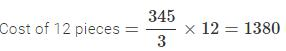QUESTION: 7

A beaker is 3/7 filled with water. Another 20 litres of water is needed to fill the beaker to its brim. What is the capacity of the beaker?

Solution:

Let capacity of beaker = x
Then,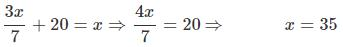QUESTION: 8

Mrs. Reeta had 17.85 m of ribbon. She cut 11 smaller pieces each of length 0.45 m from it. How many metres of ribbon were left?

Solution:

Length of smaller pieces = 11×0.45 = 4.95
Hence,

ribbon left with Reeta =17.85 − 4.95 = 12.90

QUESTION: 9

A tailor had a piece of cloth 7 m long. He cut 5 smaller pieces of cloth each 3/4 m from it. How many metres of cloth were left?

Solution: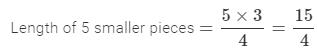∴ cloth left with tailor =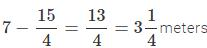QUESTION: 10

A shopkeeper mixed 4.75 kg of hazelnuts with 0.75 kg of raisins. He packed the mixture equally into 5 boxes. What is the weight of each box?

Solution:

Total weight of hazelnuts and raisins = 4.75+0.75=5.50kg
∴ weight of each box =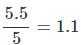QUESTION: 11

Express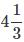hrs in seconds.

Solution: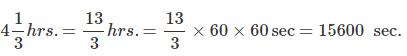QUESTION: 12

It takes 30 minutes to walk __________.

Solution:
QUESTION: 13

What goes in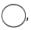2750cm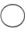28 m

Solution:
QUESTION: 14

Aman's bag is 2.14 kg lighter than Ajay bag. The weight of Ajay bag is 4.72 kg. How much does Aman bag weight?

Solution:

Weight of Aman's bag = 4.72−2.14 = 2.58

QUESTION: 15

Mary bought 450 gms of Amul Butter and 250 gms of mother dairy butter. How much butter in kg she bought?

Solution:

Total weight of butter = 450+250 = 700g = 0.7kg

QUESTION: 16

A tea pot holds about 1800 ml of tea. How many people can drink if they want 2 cups of tea having capacity of 150 ml?

Solution:

Quantity of tea that a person can drink = 2×150 = 300ml
∴ number 01 people = 1800/300 = 6

QUESTION: 17

A city tower is 1,385 feet tall with 85 stories. The residential building is 1505 feet tall with 105 stories. How much taller is the residential building than the city tower?

Solution:

1505−1385=120 feet

QUESTION: 18

Students collected bags of cloths and glass bottles. Each bag holds 21 bottles and each bottle contains 600 mi of juice. If students filled 17 bags then how much of juice did they collect?

Solution:

21×0.6×17 = 214.2

QUESTION: 19

It takes Alisha's family complete 3 days to drive to the hill station. They spend 4 days at the shore. Then drive home again. If they left home early Saturday on 5th Jan, what day and date will they return to home?

Solution:

3 days to drive to the hill station and 3 days to drive to reach home  and  spend 4 days at the shore.

Total days = 3 + 3 + 4

= 10

left home, Saturday on 5th Jan and 10 days used on the trip

Hence, came back to the home after 10 days i.e. 15th Jan, Thursday

QUESTION: 20

To circulate the information about Global Olympiad Federation, coordinator decided to paste a poster on the notice board, whose maximum vertical height is 18 inches. Coordinator wanted to know whether the height of tine poster should be 2 feet long or 40 centimeter long.

Solution: